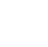Back to Chapter
Getting StartedBasic Syntax & DeclarationControl StatementsNo items found.
Function & EventsNo items found.
Array and its TypesNo items found.
OOP : Object Oriented ProgrammingNo items found.
Javascript StandardsNo items found.
HTML DOMNo items found.No items found.No items found.No items found.

# Introduction

You have learnt about looping in Javascript. Now, We will now use another Looping Syntax i.e. For Loop

## Syntax :

``` for (initialExpression; condition; incrementExpression){ // statements } ```

### Explanation :

1. The initializing expression is the initial expression that declares any variable and executes it only once.
2. The condition expression is evaluated.
3. If the value of the conditional expression is true, the loop statements execute.
4. If the value of the condition is false, the for loop terminates.
5. the incrementExpression updates the value for Next Iteration.

Flow Chart

## Example 1:

First Program is to Print "Hello World"  10 Times.

``` var i; for(i=1;i<=10;i++){ console.log("Hello world"); } //output: //Hello world //Hello world //Hello world //Hello world //Hello world //Hello world //Hello world //Hello world //Hello world //Hello world ```

Here,

We have declared a variable i

Next, We have used a For statement with :

initialExpression as i=1

condition as i≤=10

and incrementExpression as i++ i.e i=i+1

This Will be Executed 10 Times.

## Example 2:

We will make a program that gives the sum of first 10 natural numbers.

``` var sum = 0; var numbers = 10; for(i=1;i<=10;i++){ sum += i; } console.log(sum); //output: //55 ```

Explanation:

## Example 3:

Now We Will Study About Nested Loop

Nested Loop Refers to the Loop inside a Loop, you can create any number of Loop inside Any Number of Loops.

## Syntax

``` for (initialExpression; condition; incrementExpression){ //statements for (initialExpression2; condition2; incrementExpression2){ // statements2 } //statements 1 } ```

## Example 4

Suppose You Want to Print Table of Number 1,2,3,4,5

What you gonna do create a loop from 1 to 5 for table of 1 and do same for 2,3,4 and 5

But don't you think it will take a lot of time and to write the code for all the tables.

So, Is there any shortcut Method to do so??? Yes!!! Here comes the use of Nested for loop.

Let's See How it Works.

``` ```jsx var tableNo; var rowNo; for(tableNo = 1;tableNo<=5;tableNo++){ console.log("Table Of " + tableNo + " : "); for(rowNo=1;rowNo<=10;rowNo++){ console.log(tableNo + " x " + rowNo + " = " + tableNo*rowNo); } } // Output // Table Of 1 : // 1 x 1 = 1 // 1 x 2 = 2 // 1 x 3 = 3 // 1 x 4 = 4 // 1 x 5 = 5 // Table Of 2 : // 2 x 1 = 2 // 2 x 2 = 4 // 2 x 3 = 6 // 2 x 4 = 8 // 2 x 5 = 10 // Table Of 3 : // 3 x 1 = 3 // 3 x 2 = 6 // 3 x 3 = 9 // 3 x 4 = 12 // 3 x 5 = 15 // Table Of 4 : // 4 x 1 = 4 // 4 x 2 = 8 // 4 x 3 = 12 // 4 x 4 = 16 // 4 x 5 = 20 // Table Of 5 : // 5 x 1 = 5 // 5 x 2 = 10 // 5 x 3 = 15 // 5 x 4 = 20 // 5 x 5 = 25 ``` ```

Did you See How Easy It Was... That's The Power of Looping Code Short Result Long.

Let's Understand It.

Here First We have Declared 2 Variables tableNo and rowNo